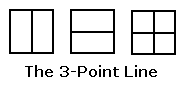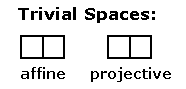# Log24

## Thursday, June 24, 2010

### Midsummer Noon

Filed under: General,Geometry — Tags: , , — m759 @ 12:00 PM

Geometry Simplified(a projective space)

The above finite projective space
is the simplest nontrivial example
of a Galois geometry (i.e., a finite
geometry with coordinates in a
finite (that is, Galois) field.)

The vertical (Euclidean) line represents a
(Galois) point, as does the horizontal line
and also the vertical-and-horizontal
cross that represents the first two points’
binary sum (i.e., symmetric difference,
if the lines are regarded as sets).

Homogeneous coordinates for the
points of this line —

(1,0), (0,1), (1,1).

Here 0 and 1 stand for the elements
of the two-element Galois field GF(2).

The 3-point line is the projective space
corresponding to the affine space
(a plane, not a line) with four points —(an affine space)

The (Galois) points of this affine plane are
not the single and combined (Euclidean)
line segments that play the role of
points in the 3-point projective line,
but rather the four subsquares
that the line segments separate.

For further details, see Galois Geometry.

There are, of course, also the trivial
two-point affine space and the corresponding
trivial one-point projective space —Here again, the points of the affine space are
represented by squares, and the point of the
projective space is represented by a line segment
separating the affine-space squares.

## No Comments

No comments yet.

RSS feed for comments on this post.

Sorry, the comment form is closed at this time.

Powered by WordPress